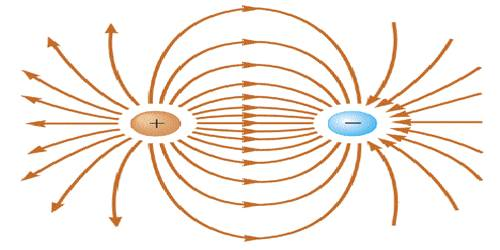# Concept of Electric Dipole

Concept of Electric Dipole

If two poles of same strength are placed very close to each other, it forms a magnetic dipole. Similarly, if two dissimilar charges of equal magnitude are placed very close to each other, it forms an electric dipole. Since the potential at any point on the perpendicular bisectors of the dipole is zero hence no work is needed to be done for transferring a positive charge along that line i.e., work done is zero. If two opposite point charges of equal magnitude are situated very close to each other, an electric dipole is formed.

As for example, in hydrogen atom there one positive proton and a negative electron. So it is an electric dipole.

The product of each of the charges of the dipole and the distance between the charges is called dipole moment. Suppose the magnitude of the charge of any of the electric dipole = q and distance between them 2l.

Dipole moment p = q x 2l. It is a vector quantity whose direction is from negative charge to positive charge.